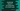# 4 JavaScript program to check if the first character of a string is in lower case or not## JavaScript program to check if the first character of a string is in lower case or not:

This post will show you how to check if the first character of a string is in lower case or not. For example, the string helloWorld starts with a lower case character but HelloWorld starts with a upper case character. We will learn different ways to check it with examples for each.

### Method 1: By comparing the first character with its lower case value:

We can simply compare the first character of a string with its lower case value. If both are equal, it means that the string starts with a lower case character.

We don’t have any method in JavaScript strings to check if the current character is in upper case or in lower case. But, we have methods to convert a charater to lowercase and also we have method to get a character at any specific index in a string.

The charAt method can be used to get a character at any specific index of a string. We can pass 0 to this method to get the first character of a string.

Similarly, we can use the toLowerCase() method to convert a character to lower case. So, we need to call charAt(0) to get the first character of a string and we can call toLowerCase() on this character to get the lower case value. If it is equal to the original character, the string starts with a lower case value.

Let’s write down the complete program:

``````const isStartingLowerCase = str => str.charAt(0) === str.charAt(0).toLowerCase();;

let givenArr = ['hello', 'Hello', 'hEllO', 'HELLO WORLD', ' hello', '', '123hello', '@#\$hello'];

givenArr.forEach(e => console.log(`\${e} => \${isStartingLowerCase(e)}`));``````

The array givenArr is an array of strings. We are using the method isStartingLowerCase to check if the first character of a string is lower case or not. The loop iterates through the strings in the array one by one and checks if the first character of each string is in lower case or not.

If you run the above program, it will print the below result:

``````hello => true
Hello => false
hEllO => true
HELLO WORLD => false
hello => true
=> true
123hello => true
@#\$hello => true``````

It failed if the string is starting with number, empty character or any other special character. It works only for alphabets.

### Method 2: Lower case the first character and append it with the rest:

This is almost similar to the above program. We can convert the first character to lower case and append it with the rest of the string and compare it with the original string. If both are equal, the string is starting with a lower case character. Else, it is not.

``````const isStartingLowerCase = str => str === str.charAt(0).toLowerCase() + str.slice(1);

let givenArr = ['hello', 'Hello', 'hEllO', 'HELLO WORLD', ' hello', '', '123hello', '@#\$hello'];

givenArr.forEach(e => console.log(`\${e} => \${isStartingLowerCase(e)}`));``````

It will print:

``````hello => true
Hello => false
hEllO => true
HELLO WORLD => false
hello => true
=> true
123hello => true
@#\$hello => true``````

The output is similar to the above example.

### Method 3: By using charCodeAt:

The charCodeAt method returns UTF-16 code unit for a character. It takes the index of the character as the parameter and returns the UTF-16 code unit for the character at the provided index. This value can be compared with 97 and 122, which are the character code values for a and z.

Let’s write down the program:

``````const isStartingLowerCase = str => str.charCodeAt(0) >= 97 && str.charCodeAt(0) <= 122;

let givenArr = ['hello', 'Hello', 'hEllO', 'HELLO WORLD', ' hello', '', '123hello', '@#\$hello'];

givenArr.forEach(e => console.log(`\${e} => \${isStartingLowerCase(e)}`));``````

It will print the correct results:

``````hello => true
Hello => false
hEllO => true
HELLO WORLD => false
hello => false
=> false
123hello => false
@#\$hello => false``````

### Method 4: By using regular expression, regex:

We can use the regular expression /[a-z]/ to match with the first character of a string. It will match only for lowercase characters. So, if the first character is a lowercase character, it will return true. Else, it will return false.

It can be used with the test() function as like below:

``````const isStartingLowerCase = str => /[a-z]/.test(str.charAt(0));

let givenArr = ['hello', 'Hello', 'hEllO', 'HELLO WORLD', ' hello', '', '123hello', '@#\$hello'];

givenArr.forEach(e => console.log(`\${e} => \${isStartingLowerCase(e)}`));``````

It will give correct results:

``````hello => true
Hello => false
hEllO => true
HELLO WORLD => false
hello => false
=> false
123hello => false
@#\$hello => false``````